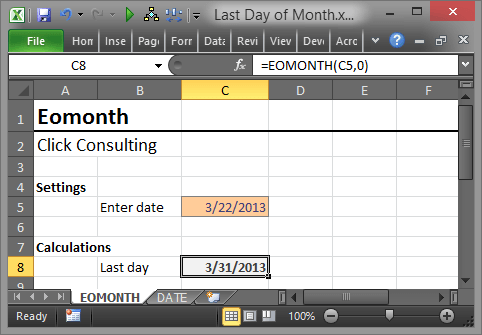# Compute the Last Day of the Month in ExcelAs you probably know, I like converting manual input cells to formula cells in Excel. To me, the more I can delegate to Excel the better off I am.  Some workbooks can benefit by automatically computing the last day of the month, eliminating one more manual input cell.

As you also know, it seems that in Excel, there are many ways to accomplish any given task. Here, I’ll explore two methods for computing the last day of a given month.

## EOMONTH

My favorite method is the EOMONTH function.  The EOMONTH function returns the end of the month (last day of the month) for any given date.## Function Arguments

There are two function arguments, the first argument is the starting date. The second argument is the number of months in the future to go, where 0 means the current month (the same month as the starting date).

For example, if cell A1 stored the date 3/22/2013, and I wanted to compute the last day of the month for the date stored in A1, or 3/31/2013, I would use:

=EOMONTH(A1,0)

If I wanted to compute the last day of the month for the following year, or 3/31/2014, I would use:

=EOMONTH(A1,12)

What is cool about this function, is that it is just as easy to compute the first day of any month by simply adding 1 to the EOMONTH result. For example, if I wanted to compute the first day of the month that follows the date in A1, or 4/1/2013, I would use:

=EOMONTH(A1,0)+1

For accounting professionals that use date driven workbooks, being able to quickly determine the last day of any given month is pretty handy.

## DATE

Another approach is by using the DATE function, with a twist. The twist is on the day argument.  So, the syntax for the function is:

=DATE(year, month, day)

When you use 0 for the day, it goes back one day, to the last day of the previous month. The following function returns the date of 7/1/2013.

=DATE(2013,7,1)

If we use 0 for the day argument rather than 1, then the prior day is returned. For example, the following formula returns the date of 6/30/2013:

=DATE(2013,7,0)

If you had a date in cell A1, you could use the MONTH and YEAR functions to pull the month and year out. If you wanted to determine the last day of the month prior to the date in A1, you could use the following formula:

=DATE(YEAR(A1),MONTH(A1),0)

Or, if you wanted to compute the last day of the month of the date in A1:

=DATE(YEAR(A1),MONTH(A1)+1,0)

So, these are two pretty handy ways to determine the last day of the month.

Excel rules!

Here is a workbook Last Day of Month that uses these functions.

Note: Depending on which version of Excel you have, you may need to activate the Analysis ToolPak before getting access to the EOMONTH function.

Get a quick email notice when a new Excel article is available

• This field is for validation purposes and should be left unchanged.